Foxtable(狐表)用户栏目专家坐堂 → 数据平均问题

共有928人关注过本帖树形打印复制链接

# 主题：数据平均问题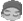11楼 | 信息 | 搜索 | 邮箱 | 主页 | UC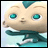加好友发短信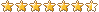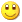Post By：2022/8/11 14:21:00 [只看该作者]

 是的哦，看漏眼了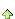12楼 | 信息 | 搜索 | 邮箱 | 主页 | UC加好友发短信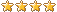Post By：2022/8/12 10:11:00 [只看该作者]

 Dim avg1 As Decimal = tb5.Compute("avg(分班成绩)","预分班='" & r("班级") & "'")这样就可以了13楼 | 信息 | 搜索 | 邮箱 | 主页 | UC加好友发短信Post By：2022/8/13 10:24:00 [只看该作者]

 请问老师，我想将表按性别，成绩排序后，按记录依次按8个一组，随机将1-8这8个数字填到每个组的8个数据中去，这样可实现相对均衡，这该怎么处理14楼 | 信息 | 搜索 | 邮箱 | 主页 | UC加好友发短信Post By：2022/8/13 10:49:00 [只看该作者]

 Dim lst As New List(Of Integer)For i As Integer = 1 To 15 '模拟学生编号1至15号    lst.Add(i)NextDim cnt As Integer = lst.CountFor i As Integer = 0 To cnt - 1 Step 8 '8个一组    Dim lst2 As New List(Of Integer)    For m As Integer = 1 To 8 '分配的8个数字        lst2.Add(m)    Next    Output.Show("----------")    Dim n As Integer = Math.Min(i + 7, cnt - 1)    For j As Integer = i To n        Dim k As Integer = Rand.Next(0, lst2.count)        Output.Show("编号=" & j & ",分配=" & lst2(k))        lst2.RemoveAt(k)    Next Next15楼 | 信息 | 搜索 | 邮箱 | 主页 | UC加好友发短信Post By：2022/8/14 22:27:00 [只看该作者]

 Dim tb5 As Table= Tables("学生信息A_table5")Dim tb10 As Table= Tables("学生信息A_table10")Dim cb2 As String = e.Form.Controls("combobox2").textDim bs As Integer= e.Form.Controls("combobox3").textDim add As Boolean=Truetb5.sort="性别,分班成绩 desc"Dim cnt As Integer = tb5.Rows.countFor i As Integer = 0 To cnt - 1 Step bs '8个一组    Dim lst2 As New List(Of Integer)    For m As Integer = 1 To bs '分配的8个数字        lst2.Add(m)    Next        Dim n As Integer = Math.Min(i + bs-1, cnt - 1)    For j As Integer = i To n        Dim k As Integer = Rand.Next(0, lst2.count)        tb5.Rows(j)("预分班")= cb2 & lst2(k) & "班"        lst2.RemoveAt(k)    Next 0Next 老师，我这样用就可以了，也给大家分享下16楼 | 信息 | 搜索 | 邮箱 | 主页 | UC加好友发短信Post By：2022/8/14 22:31:00 [只看该作者]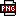此主题相关图片如下：qq图片20220814222830.png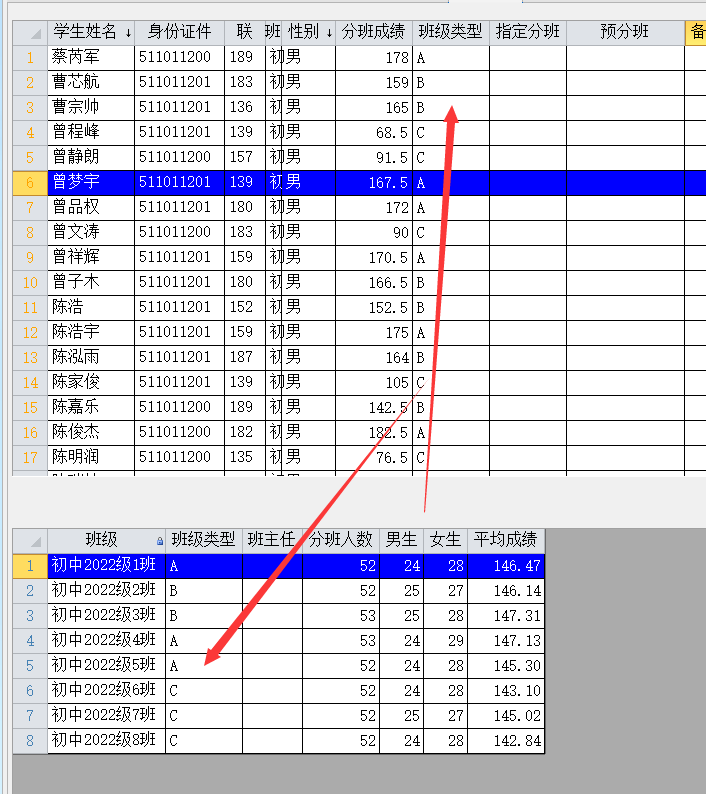现在我想在上面的基础上增加一个功能，就是班级有三类A,B,C类班，学生先按成绩分为A,B,C三类，现在要将A类学生均衡分班A类班级，这又怎么来改整来，谢谢17楼 | 信息 | 搜索 | 邮箱 | 主页 | UC加好友发短信Post By：2022/8/15 8:35:00 [只看该作者]

 就是多套一个循环先筛选出成绩为A类的学生，按15楼的方法处理；接着筛选B类的学生，.........18楼 | 信息 | 搜索 | 邮箱 | 主页 | UC加好友发短信Post By：2022/8/16 9:41:00 [只看该作者]

 Dim tb5 As Table= Tables("学生信息A_table5")Dim tb10 As Table= Tables("学生信息A_table10")Dim cb2 As String = e.Form.Controls("combobox2").textDim add As Boolean=Truetb5.sort="性别,分班成绩 desc"Dim bjnxs As List(Of String) = tb10.DataTable.GetValues("班级类型")For Each bjnx  As String In bjnxs    Dim bjlist As New List(Of String)    For Each r As Row In tb10.Rows        If r("班级类型")=bjnx            bjlist.add(r("班级"))        End If    Next    tb5.Filter="班级类型='" & bjnx & "'"    Dim cnt As Integer =tb5.Rows.count    Dim bs As Integer = bjlist.Count    For i As Integer = 0 To cnt - 1 Step bs '8个一组        Dim lst2 As New List(Of Integer)        For m As Integer = 1 To bs '分配的8个数字            lst2.Add(m)        Next        Dim n As Integer = Math.Min(i + bs-1, cnt - 1)        For j As Integer = i To n            Dim k As Integer = Rand.Next(0, lst2.count)            tb5.Rows(j)("预分班")= bjlist(k)            lst2.RemoveAt(k)        Next    Next    Nexttb5.Filter="" 老师,请问我这样改后为什么同类班人数没均衡,请帮看下哪里有问题,谢谢19楼 | 信息 | 搜索 | 邮箱 | 主页 | UC加好友发短信Post By：2022/8/16 9:54:00 [只看该作者]

 没均衡具体什么现象，截图说明一下20楼 | 信息 | 搜索 | 邮箱 | 主页 | UC加好友发短信Post By：2022/8/16 10:03:00 [只看该作者]此主题相关图片如下：qq图片20220816100154.png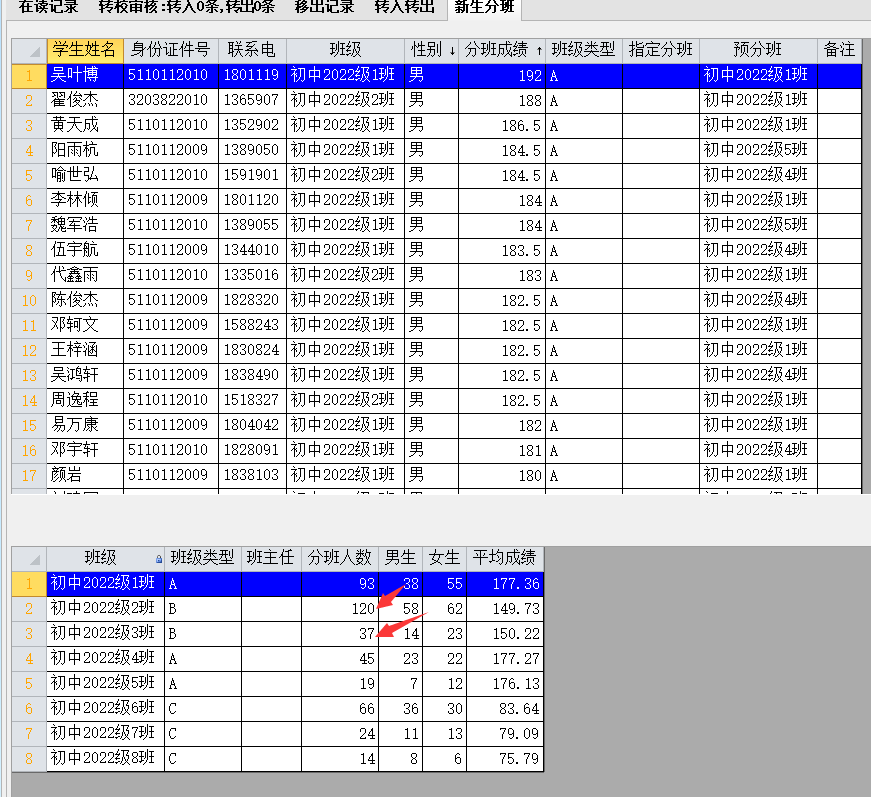我设想的是同类班级人如A类3个班,在人数,成绩,性别上随机均衡,现在主要存在的是人数就不均衡 [此贴子已经被作者于2022/8/16 10:03:30编辑过]总数 21 上一页 1 2 3 下一页